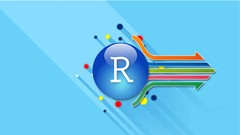--># Hands-On R programming with Statistics for Data science

Hands-On R programming with Statistics for Data science, Learn applied statistics and data manipulation using real case studies
NEW
Created by Hands-On System
English
English [Auto-generated]

PREVIEW THIS COURSE - GET COUPON CODE

What you'll learn
• Learn R programming from scratch
• Use of R Studio
• Principles of programming
• Concept of vectors in R
• Data types in R
• Know the use of while() and for()
• Build and use matrices in R
• Use matrix() function, learn rbind() and cbind()
• Install packages in R
• Understand the Normal distribution
• Practice working with statistical data in R
• R functions
• Hypothesis testing for mean
• Multiple Linear Regression
• Logistic Regression
• Time Series Analysis
• Cluster analysis
• Factor Analysis
• Decision Tree
• Sentimental Analysis